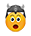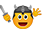## A math problem for the wise

58 Replies
24 June, 2019, 6:50 AM UTC

Hello Lords!

We have an exercise for you that seems easy only at first glance. Can you solve it and explain your answer?UTC +7:00
1
User
24 June, 2019, 7:26 AM UTC

The answer is 1 My Lord.

9 / 3(2+1) =

9 / 3(3) =

9/9 = 1

You must firstly perform calculations in parenthesis (2+1)=3, and then perform the multiplication 3*(3)=9 Lastly solve the equation by dividing 9/9, thus giving the answer to the equation as 1

Queen Sparta

Now Lord give me my Gold!

UTC +0:00
1
User
24 June, 2019, 10:36 AM UTC

First you must fearlessly tame the numbers in the cage thus (2+1) = (3)

Then you strike the caged 3 three times and by blue-smoked magic this makes 9

Next you fearlessly stand the nines together divided by the slim trembling / and whisper the magic chant, "There can be only 1"

And thus the answer is 1.
UTC +0:00
1
User
24 June, 2019, 11:07 AM UTC

The fearless Order of the Operations has rules. Brackets must be dealt to first, leaving the expression 9 divided by 3 times 3 (as wise members of the order know, in algebra, beware of the invisible multiplication signs).

When all smokescreens are removed and only division and multiplication is left, then charge thy enemy in a calculated fashion, from left to right. This will lead thee to the correct answer: 9.
UTC +10:00
2
User
24 June, 2019, 12:31 PM UTCUTC +0:00
1
User
24 June, 2019, 2:11 PM UTC

*please excuse my dear aunt sally

2+1=3

3*3=9

9/9=1

UTC +0:00
1
User
24 June, 2019, 7:46 PM UTC
Add brackets first; then it will be 9 divided by 9 = 1.
UTC +0:00
0
User
25 June, 2019, 5:42 AM UTC
9:3(2+1) = 3(3) = 9
UTC +1:00
0
User
25 June, 2019, 9:37 AM UTC
9 / 3 * 3 = 9
UTC +0:00
1
User
25 June, 2019, 3:24 PM UTC

Hello!

This is  a fraction written in different way, so if we write it like a fraction, we get

9               9             9

--------- =  -------- = -------- =  1

3(2+1)        3x3           9UTC +0:00
1
User
25 June, 2019, 6:20 PM UTC
It`s 9 the answer. First we solve what is in the brackets and  what is left is 9/3*3 . In this position we go from left to right 9/3=3 and 3*3=9
UTC +0:00
0
User
25 June, 2019, 10:27 PM UTC

The solution is 9 !

as division operations are  neither commutative nor associative it is not allowed to simply switch terms in a series of alternating divisions and multiplications.

One then always has to go from left to right:  a : b * c = (a : b) * c

Therefore after the brackets are of course solved first:

9 : 3 * (1+2) = 9 : 3 * 3 = (9:3) * 3 = 3 * 3 = 9

UTC +0:00
0
User
27 June, 2019, 12:10 AM UTC

parenthesis first.

then multiplication and division left to right.

then addition and subtraction left to right

9 / 3 (1+2)  can also be written as

9 / 3 * (1+2) so therefore it becomes

9 / 3 * 3 so left to right gives us

3 * 3 which is

9
UTC +0:00
1
User
27 June, 2019, 10:03 AM UTC

9 / 3 (2 +1) =

1st step,  resolve the operation in parenthesis, (2+1) = 3

the following operation can be written : 9 / 3 x 3

multiplication and division are then priority, but multiplication and division are of the same family, so in the presence of these 2 operations, always carry out the first operation starting with the left

9 / 3 = 3 and 3 x 3 = 9

the solution is 9

UTC +2:00
0
User
27 June, 2019, 10:09 AM UTC

Always perform whats inside the parenthesis first, then work left to right, and what's left in parenthesis is implied multiplication, at least this is what my 4th grade math teacher said back in '75
UTC +0:00
0
User
27 June, 2019, 11:40 AM UTC

= 9 / 3(2+1)

= 9 / 3(3)

= 9 / 3x3

= 9 / 9

=1
UTC +3:00
0
User
27 June, 2019, 12:24 PM UTC

Hello

9:3 (2+1) = 9 !

(2+1) = 3

9:3 = 3

and 3x3 = 9 !

UTC +0:00
0
User
27 June, 2019, 1:01 PM UTC
UTC +0:00
0
User
27 June, 2019, 1:42 PM UTC

1+1+1+1+1+1+1+1+1=9

UTC +0:00
0
User
27 June, 2019, 1:50 PM UTC

It's a linguistic maths problem.

(9/3)*3 = 9 or 9/(3*3) = 1

Now we have the 3*3 as 3(3) Some consider this as an implicit bracket in terms of order of precedence. Others go with it being a multiplication, and hence you should work from left to right. It is deliberately ambiguous.
UTC +0:00
0
7143158 users registered; 139422 topics; 495839 posts; our newest member:JolyLaunch